Matematicas Visuales | Resources: The golden rectangle and the icosahedronResources: The golden rectangle and the icosahedron

Very nice construction of an icosahedron. You only need three golden rectangles.To learn how to draw a golden rectangle is a beautiful experience. The golden number is related with the regular pentagon: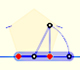You can draw a regular pentagon given one of its sides constructing the golden ratio with ruler and compass.

I think that the best material to build this model is wood. But it is very simple to build one using cardboard. You can download and print this drawing of several golden rectangles: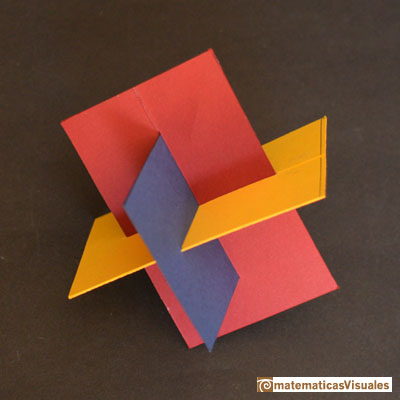Now we can build one model using wood or DM. This example has detachable parts: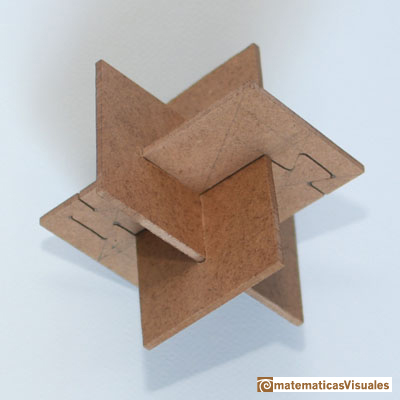We start drawing: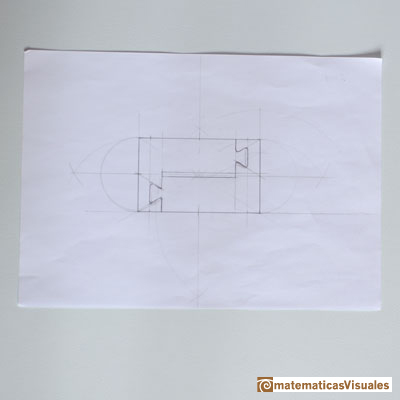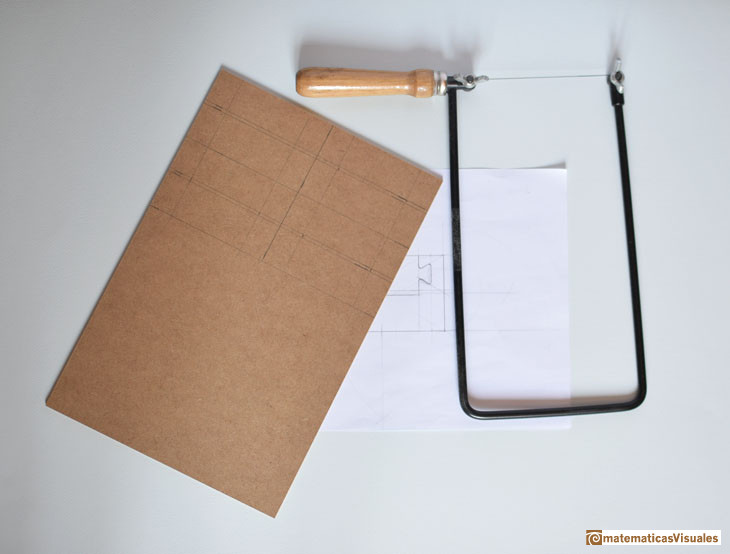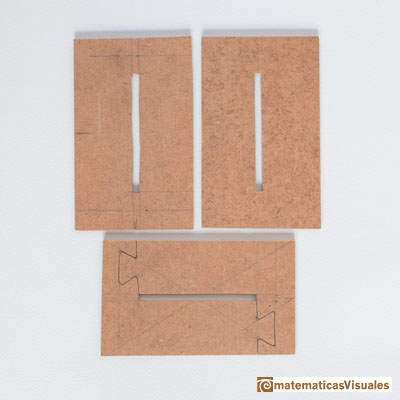We can use different materials:If you have access to a laser cutting machine you can reproduce the model as many times as you want. The result is very accurate. Sara San Gregorio, who develops the proyect Microarquitectura in MediaLab-Prado in Madrid, produced a digital file with the drawing and cut this model: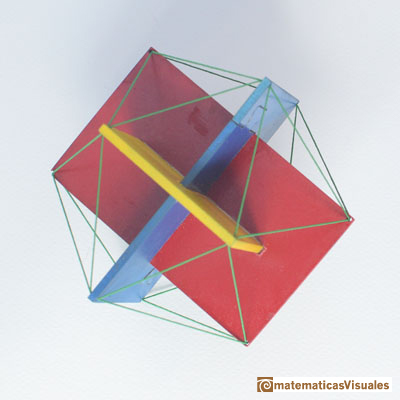TO THINK A LITTLE

All the triangles are equilateral?You can se the relation with the diagonal of a pentagon.

This construction is related with the Borromean Rings.

Borromean Rings made using rubber balloons. Inspired in the logo of the International Mathematical Union (IMU) designed by John Sullivan (The Borromean Rings: a new logo for the IMU)It is very easy to build an icosahedron using cardboard. Durer showed us how to draw its plane net.REFERENCES

H.S.M. Coxeter - 'Introduction to Geometry', Wyley.
Hilber y Cohn-Vossen - "Geometry and the Imagination", AMS Chelsea Pub.
Magnus Wenninger - 'Polyhedron Models', Cambridge University Press.
Hugo Steinhaus - Mathematical Snapshots - Oxford University Press - Third Edition (p. 197)
Peter R. Cromwell - 'Polyhedra', Cambridge University Press, 1999.
H.Martin Cundy and A.P. Rollet, 'Mathematical Models', Oxford University Press, Second Edition, 1961 (p. 87).
W.W. Rouse Ball and H.S.M. Coxeter - 'Matematical Recreations & Essays', The MacMillan Company, 1947.Simple technique to build polyhedra gluing discs made of cardboard or paper.Italian designer Bruno Munari conceived 'Acona Biconbi' as a work of sculpture. It is also a beautiful game to play with colors and shapes.Material for a session about polyhedra (Zaragoza, 9th May 2014). Simple techniques to build polyhedra like the tetrahedron, octahedron, the cuboctahedron and the rhombic dodecahedron. We can build a box that is a rhombic dodecahedron.Material for a session about polyhedra (Zaragoza, 7th November 2014). We study the octahedron and the tetrahedron and their volumes. The truncated octahedron helps us to this task. We build a cubic box with cardboard and an origami tetrahedron.Material for a session about polyhedra (Zaragoza, 23rd Octuber 2015) . Building a cube with cardboard and an origami octahedron.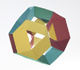Material for a session about polyhedra (Zaragoza, 21st October 2016). Instructions to build several geometric bodies.Microarquitectura is a construction game developed by Sara San Gregorio. You can play and build a lot of structures modelled on polyhedra.The twelve vertices of an icosahedron lie in three golden rectangles. Then we can calculate the volume of an icosahedronThe volume of a tetrahedron is one third of the prism that contains it.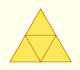The first drawing of a plane net of a regular tetrahedron was published by Dürer in his book 'Underweysung der Messung' ('Four Books of Measurement'), published in 1525 .Some properties of this platonic solid and how it is related to the golden ratio. Constructing dodecahedra using different techniques.From Euclid's definition of the division of a segment into its extreme and mean ratio we introduce a property of golden rectangles and we deduce the equation and the value of the golden ratio.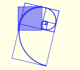The golden spiral is a good approximation of an equiangular spiral.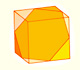We can cut in half a cube by a plane and get a section that is a regular hexagon. Using eight of this pieces we can made a truncated octahedron.Using eight half cubes we can make a truncated octahedron. The cube tesselate the space an so do the truncated octahedron. We can calculate the volume of a truncated octahedron.Leonardo da Vinci made several drawings of polyhedra for Luca Pacioli's book 'De divina proportione'. Here we can see an adaptation of the truncated octahedron.The truncated octahedron is an Archimedean solid. It has 8 regular hexagonal faces and 6 square faces. Its volume can be calculated knowing the volume of an octahedron.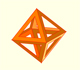The volume of an octahedron is four times the volume of a tetrahedron. It is easy to calculate and then we can get the volume of a tetrahedron.